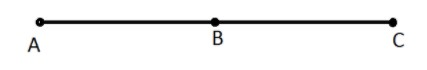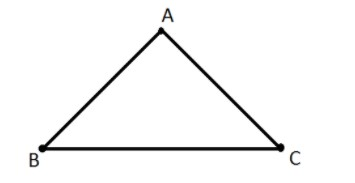QuestionAnswers

# A triangle is formed by joining three ………. points.A.CollinearB.Non collinearC.EqualD.None of theseVerified
128.7k+ views
Hint: A triangle is a type of polygon, which has three sides and the two sides are joined end to end is called the vertex of the triangle. An angle is formed between two sides.

Complete step by step solution:
The points lying on the same line are called collinear pointsWhereas,
The points that do not lie on the same line are called non-collinear points.Points A, B and C are non-collinear points
$\to$The sum of the angles of a triangle is always $180$degree
$\to$The exterior angles of triangle always add up to ${360^0}$degrees
$\to$The shortest side is always opposite the smallest interior angle. Similarly, the longest side is always opposite the largest interior angle.
Area of triangle: -

Area of triangle $=$ Half of product of base and height
$Area = \dfrac{1}{2} \times Base \times Height$
If ABC is a triangle, then it is denoted as $\vartriangle ABC$ where A, B, and C are the vertices of the triangle. A triangle is a two-dimensional shape in Euclidean geometry, which is seen as three non-collinear points in a unique plane.

Hence a triangle is formed by joining three non-collinear points.

Note: A triangle has 6 elements. Three sides and three angles. Depending on the length of the sides and angles of the triangle we can decide what type of triangle it is.